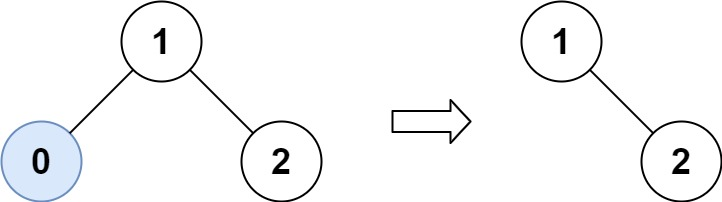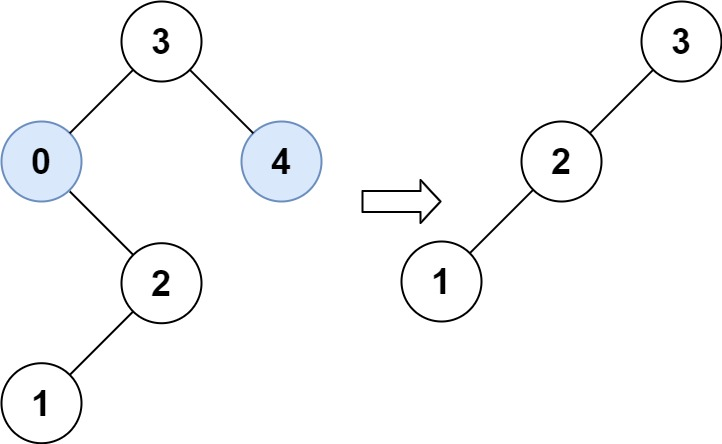| English | 简体中文 |

# 669. Trim a Binary Search Tree

## Description

Given the root of a binary search tree and the lowest and highest boundaries as low and high, trim the tree so that all its elements lies in [low, high]. Trimming the tree should not change the relative structure of the elements that will remain in the tree (i.e., any node's descendant should remain a descendant). It can be proven that there is a unique answer.

Return the root of the trimmed binary search tree. Note that the root may change depending on the given bounds.

Example 1:Input: root = [1,0,2], low = 1, high = 2
Output: [1,null,2]


Example 2:Input: root = [3,0,4,null,2,null,null,1], low = 1, high = 3
Output: [3,2,null,1]


Constraints:

• The number of nodes in the tree in the range [1, 104].
• 0 <= Node.val <= 104
• The value of each node in the tree is unique.
• root is guaranteed to be a valid binary search tree.
• 0 <= low <= high <= 104